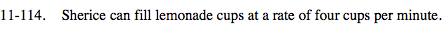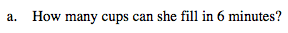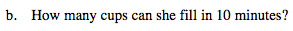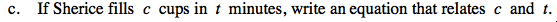### Home > MC2 > Chapter 11 > Lesson 11.3.3 > Problem11-114

11-114.To find the number of cups filled in a certain amount of time, multiply the rate by the time.

$\left(\frac{4\; \text{cups}}{\text{minute}}\right)\cdot (6\; \text{minutes})$

24 cupsSee the help for part (a).Notice that in parts (a) and (b), the rate must be multiplied by time (t) in order to find the number of cups (c).

c = 4t